Advanced Database Management System - Tutorials and Notes: Bigram probability estimate of a word sequence

## Bigram Model - Probability Calculation - Example Problem

Solved Example:

Let us solve a small example to better understand the Bigram model. For this we need a corpus and the test data. Let us assume that the following is a small corpus;

Training corpus:
<s> I am from Vellore </s>
<s> I am a teacher </s>
<s> students are good and are from various cities</s>
<s> students from Vellore do engineering</s>

Test data:
<s> students are from Vellore </s>

Let us find the Bigram probability of the given test sentence. I explained the solution in two methods, just for the sake of understanding. the second method is the formal way of calculating the bigram probability of a sequence of words.

Method 1
As per the Bigram model, the test sentence can be expanded as follows to estimate the bigram probability;
P(<s> students are from Vellore </s>)
= P(students | <s>) * P(are | students) * P(from | are)
* P(Vellore | from) *    P(</s> | Vellore)

To estimate bigram probabilities, we can use the following equation;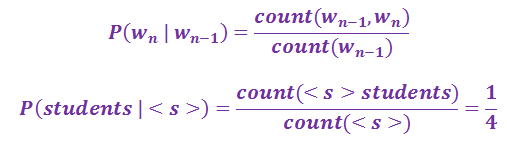[Hint – count of sentence start (<s>) = 4, count of string <s> students = 1]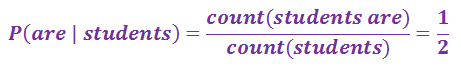[Hint – count of word students = 2, count of string students are = 1]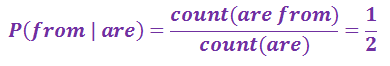[Hint – count of word are = 2, count of string are from = 1]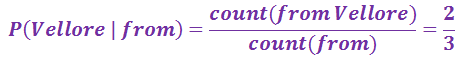[Hint – count of word from = 3, count of string from Vellore = 2]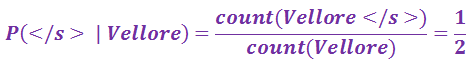[Hint – count of word Vellore = 2, count of string Vellore </s> = 1]
P(<s> students are from Vellore </s>)
= P(students | <s>) * P(are | students) * P(from | are)
* P(Vellore | from) * P(</s> | Vellore)
= 1/4 * 1/2 * 1/2 * 2/3 * 1/2 = 0.0208

Method 2
Formal way of estimating the bigram probability of a word sequence:
The bigram probabilities of the test sentence can be calculated by constructing Unigram and bigram probability count matrices and bigram probability matrix as follows;
Unigram count matrix
 students are from Vellore 4 2 2 3 2

Bigram count matrix
 wn students are from Vellore wn-1 1 0 0 0 0 students 0 1 1 0 0 are 0 0 1 0 0 from 0 0 0 2 0 Vellore 0 0 0 0 1

Bigram probability matrix (normalized by unigram counts)
 wn students are from Vellore wn-1 1/4 0/4 0/4 0/4 0/4 students 0/2 1/2 1/2 0/2 0/2 are 0/2 0/2 1/2 0/2 0/2 from 0/3 0/3 0/3 2/3 0/3 Vellore 0/2 0/2 0/2 0/2 1/2

P(<s> students are from Vellore </s>)
= P(students | <s>) * P(are | students) * P(from | are)
* P(Vellore | from) * P(</s> | Vellore)
= 1/4 * 1/2 * 1/2 * 2/3 * 1/2 = 0.0208

The probability of the test sentence as per the bigram model is 0.0208.

----------------------------------------------------------------------------------------------------------